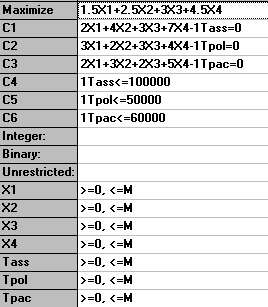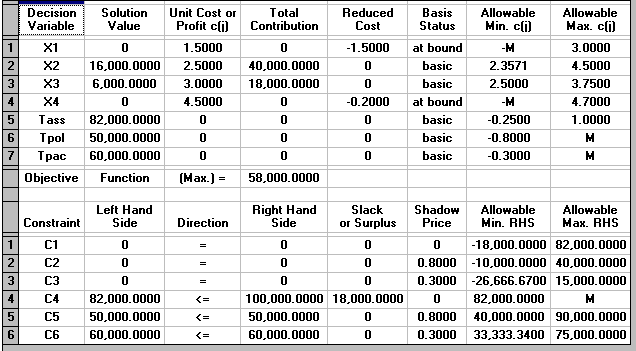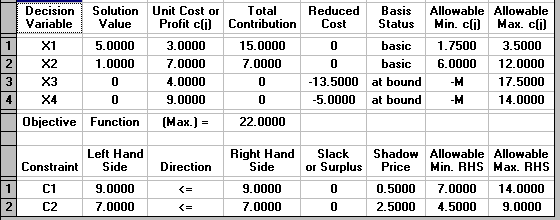# OR-Notes

## J E Beasley

OR-Notes are a series of introductory notes on topics that fall under the broad heading of the field of operations research (OR). They were originally used by me in an introductory OR course I give at Imperial College. They are now available for use by any students and teachers interested in OR subject to the following conditions.

A full list of the topics available in OR-Notes can be found here.

#### Linear programming - sensitivity analysis - using QSB

Recall the production planning problem concerned with four variants of the same product which we formulated before as an LP. To remind you of it we repeat below the problem and our formulation of it.

#### Production planning problem

A company manufactures four variants of the same product and in the final part of the manufacturing process there are assembly, polishing and packing operations. For each variant the time required for these operations is shown below (in minutes) as is the profit per unit sold.

```                Assembly      Polish    Pack        Profit (£)
Variant 1       2             3         2           1.50
2       4             2         3           2.50
3       3             3         2           3.00
4       7             4         5           4.50
```
• Given the current state of the labour force the company estimate that, each year, they have 100000 minutes of assembly time, 50000 minutes of polishing time and 60000 minutes of packing time available. How many of each variant should the company make per year and what is the associated profit?
• Suppose now that the company is free to decide how much time to devote to each of the three operations (assembly, polishing and packing) within the total allowable time of 210000 (= 100000 + 50000 + 60000) minutes. How many of each variant should the company make per year and what is the associated profit?

#### Variables

Let:

xi be the number of units of variant i (i=1,2,3,4) made per year

Tass be the number of minutes used in assembly per year
Tpol be the number of minutes used in polishing per year
Tpac be the number of minutes used in packing per year

where xi >= 0 i=1,2,3,4 and Tass, Tpol, Tpac >= 0

#### Constraints

(a) operation time definition

Tass = 2x1 + 4x2 + 3x3 + 7x4 (assembly)
Tpol = 3x1 + 2x2 + 3x3 + 4x4 (polish)
Tpac = 2x1 + 3x2 + 2x3 + 5x4 (pack)

(b) operation time limits

The operation time limits depend upon the situation being considered. In the first situation, where the maximum time that can be spent on each operation is specified, we simply have:

Tass <= 100000 (assembly)
Tpol <= 50000 (polish)
Tpac <= 60000 (pack)

In the second situation, where the only limitation is on the total time spent on all operations, we simply have:

Tass + Tpol + Tpac <= 210000 (total time)

#### Objective

Presumably to maximise profit - hence we have

maximise 1.5x1 + 2.5x2 + 3.0x3 + 4.5x4

which gives us the complete formulation of the problem.

A summary of the input to the computer package for the first situation considered in the question (maximum time that can be spent on each operation specified) is shown below.The solution to this problem is also shown below.We can see that the optimal solution to the LP has value 58000 (£) and that Tass=82000, Tpol=50000, Tpac=60000, X1=0, X2=16000, X3=6000 and X4=0.

This then is the LP solution - but it turns out that the simplex algorithm (as a by-product of solving the LP) gives some useful information. This information relates to:

• changing the objective function coefficient for a variable
• forcing a variable which is currently zero to be non-zero
• changing the right-hand side of a constraint.

We deal with each of these in turn, and note here that the analysis presented below ONLY applies for a single change, if two or more things change then we effectively need to resolve the LP.

• suppose we vary the coefficient of X2 in the objective function. How will the LP optimal solution change?

Currently X1=0, X2=16000, X3=6000 and X4=0. The Allowable Min/Max c(i) columns above tell us that, provided the coefficient of X2 in the objective function lies between 2.3571 and 4.50, the values of the variables in the optimal LP solution will remain unchanged. Note though that the actual optimal solution value will change.

In terms of the original problem we are effectively saying that the decision to produce 16000 of variant 2 and 6000 of variant 3 remains optimal even if the profit per unit on variant 2 is not actually 2.5 (but lies in the range 2.3571 to 4.50).

Similar conclusions can be drawn about X1, X3 and X4.

In terms of the underlying simplex algorithm this arises because the current simplex basic solution (vertex of the feasible region) remains optimal provided the coefficient of X2 in the objective function lies between 2.3571 and 4.50.

• for the variables, the Reduced Cost column gives us, for each variable which is currently zero (X1 and X4), an estimate of how much the objective function will change if we make that variable non-zero.

Hence we have the table

```Variable                             X1      X4
Opportunity Cost                     1.5     0.2
New value (= or >=)                  X1=A    X4=B
or X1>=A   X4>=B
Estimated objective function change  1.5A    0.2B
```

The objective function will always get worse (go down if we have a maximisation problem, go up if we have a minimisation problem) by at least this estimate. The larger A or B are the more inaccurate this estimate is of the exact change that would occur if we were to resolve the LP with the corresponding constraint for the new value of X1 or X4 added.

Hence if exactly 100 of variant one were to be produced what would be your estimate of the new objective function value?

Note here that the value in the Reduced Cost column for a variable is often called the "opportunity cost" for the variable.

Note here than an alternative (and equally valid) interpretation of the reduced cost is the amount by which the objective function coefficient for a variable needs to change before that variable will become non-zero.

Hence for variable X1 the objective function needs to change by 1.5 (increase since we are maximising) before that variable becomes non-zero. In other words, referring back to our original situation, the profit per unit on variant 1 would need to need to increase by 1.5 before it would be profitable to produce any of variant 1. Similarly the profit per unit on variant 4 would need to increase by 0.2 before it would be profitable to produce any of variant 4.

• for each constraint the column headed Shadow Price tells us exactly how much the objective function will change if we change the right-hand side of the corresponding constraint within the limits given in the Allowable Min/Max RHS column.

Hence we can form the table

```Constraint                        Assembly    Polish    Pack
Opportunity Cost (ignore sign)    0           0.80      0.30
Change in right-hand side         a           b         c
Objective function change         0           0.80b     0.30c
Lower limit for right-hand side   82000       40000     33333.34
Current value for right-hand side 100000      50000     60000
Upper limit for right-hand side   -           90000     75000```

For example for the polish constraint, provided the right-hand side of that constraint remains between 40000 and 90000 the objective function change will be exactly 0.80[change in right-hand side from 50000].

The direction of the change in the objective function (up or down) depends upon the direction of the change in the right-hand side of the constraint and the nature of the objective (maximise or minimise).

To decide whether the objective function will go up or down use:

• constraint more (less) restrictive after change in right-hand side implies objective function worse (better)
• if objective is maximise (minimise) then worse means down (up), better means up (down)

Hence

• if you had an extra 100 hours to which operation would you assign it?
• if you had to take 50 hours away from polishing or packing which one would you choose?
• what would the new objective function value be in these two cases?

The value in the column headed Shadow Price for a constraint is often called the "marginal value" or "dual value" for that constraint.

Note that, as would seem logical, if the constraint is loose the shadow price is zero (as if the constraint is loose a small change in the right-hand side cannot alter the optimal solution).

• Different LP packages have different formats for input/output but the same information as discussed above is still obtained.
• You may have found the above confusing. Essentially the interpretation of LP output is something that comes with practice.
• Much of the information obtainable (as discussed above) as a by-product of the solution of the LP problem can be useful to management in estimating the effect of changes (e.g. changes in costs, production capacities, etc) without going to the hassle/expense of resolving the LP.
• This sensitivity information gives us a measure of how robust the solution is i.e. how sensitive it is to changes in input data.

Note here that, as mentioned above, the analysis given above relating to:

• changing the objective function coefficient for a variable; and
• forcing a variable which is currently zero to be non-zero; and
• changing the right-hand side of a constraint

is only valid for a single change. If two (or more) changes are made the situation becomes more complex and it becomes advisable to resolve the LP.

#### Linear programming sensitivity example

Consider the linear program:

`maximise `
`3x1 + 7x2 + 4x3 + 9x4`
`subject to `
```x1 + 4x2 + 5x3 + 8x4 <= 9    (1)
x1 + 2x2 + 6x3 + 4x4 <= 7    (2)
xi >= 0 i=1,2,3,4 ```

Solve this linear program using the computer package.

• what are the values of the variables in the optimal solution?
• what is the optimal objective function value?
• which constraints are tight?
• what would you estimate the objective function would change to if:
• we change the right-hand side of constraint (1) to 10
• we change the right-hand side of constraint (2) to 6.5
• we add to the linear program the constraint x3 = 0.7

Solving the problem using the package the solution is:

``

Reading from the printout given above we have:

• the variable values are X1=5, X2=1, X3=0, X4=0
• the optimal objective function value is 22.0
• both constraints are tight (have no slack or surplus). Note here that the (implicit) constraints ensuring that the variables are non-negative (xi>=0 i=1,2,3,4) are (by convention) not considered in deciding which constraints are tight.
• objective function change = (10-9) x 0.5 = 0.5. Since the constraint is less restrictive the objective function will get better. Hence as we have a maximisation problem it will increase. Referring to the Allowable Min/Max RHS column we see that the new value (10) of the right-hand side of constraint (1) is within the limits specified there so that the new value of the objective function will be exactly 22.0 + 0.5 = 22.5
• objective function change = (7-6.5) x 2.5 = 1.25. Since we are making the constraint more restrictive the objective function will get worse. Hence as we have a maximisation problem it will decrease. As for (1) above the new value of the right-hand side of constraint (2) is within the limits in the Minimum/Maximum RHS column and so the new value of the objective function will be exactly 22.0 - 1.25 = 20.75
• objective function change = 0.7 x 13.5 = 9.45. The objective function will get worse (decrease) since changing any variable which is zero at the linear programming optimum to a non-zero value always makes the objective function worse. We estimate that it will decrease to 22.0 - 9.45 = 12.55. Note that the value calculated here is only an estimate of the change in the objective function value. The actual change may be different from the estimate (but will always be >= this estimate).

Note that we can, if we wish, explicitly enter the four constraints xi>=0 i=1,2,3,4. Although this is unnecessary (since the package automatically assumes that each variable is >=0) it is not incorrect. However, it may alter some of the solution figures - in particular the Reduced Cost figures may be different. This illustrates that such figures are not necessarily uniquely defined at the linear programming optimal solution.